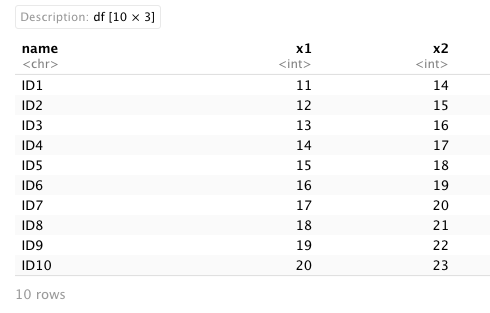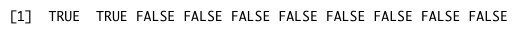# Check if Value is within a Range in R (3 Examples)

This tutorial shows how to check if a value is within a range in the R programming language.

The table of content is structured as follows:

Let’s see how this can be done.

## Data Sample

For this tutorial, we will use the following data as a basis:

```# Create example data.frame
data_sample <- data.frame(name = paste0("ID",1:10),
x1 = 11:20,
x2 = 14:23)

# Print example data.frame
data_sample```## Example 1: Find Value in a Range Using between() Function of data.table Package

We can check whether a value is within a range easily using the between() function, offered by the data.table package:

First, we have to install and load data.table:

```install.packages("data.table")
library(data.table)```

Next, we can test if a specific number is within a range. In this example, we’ll check if the value 12 lies within the ranges of the rows in the columns x1 and x2:

```value <- 12
data.table::between(value,
data_sample\$x1,
data_sample\$x2)```As we can see in our output, the between() function allows us to check whether a numeric value falls in a range or not. Thus, this function returns a list of TRUE or FALSE values: the function returns TRUE when the value we defined is between the range we specified, and FALSE when it doesn’t.

Note: I have specified the data.table package explicitly when applying the between function, since the dplyr package also contains a function called between.

## Example 2: Check if Value is within Range Using with() Function of Base R

Another solution to check if a value is within a range is using the with() function:

```with(data_sample,
x1<= value & x2 >= value)```The output we obtain using the with() function is the same as the one we obtained in the previous example. This function also gives us a vector of TRUE and FALSE values depending on if the value is within the range.

## Example 3: Check if Value is within Range Using mutate() Function of dplyr Package

The mutate() function from the dplyr package also allows us to check if a value is between a range.

First, we have to install and load dplyr:

```install.packages("dplyr")
library(dplyr)```

Next, we can apply the mutate function of the dplyr package as shown below:

```data_sample %>%
mutate(in_Range = value >= x1 & value <= x2)```The dplyr mutate() function adds a column to our data frame specifying if the value is in range (TRUE) or not (FALSE). As we can see, our output with the mutate() function fits our previous outputs.

These three functions help us to determine if a value is within a range in a dataset between columns, but can also be used to check if a value is within a range in a vector or a group of numbers.

## Video, Further Resources & Summary

Do you need more explanations on how to check if a value is between two other values? Then you should have a look at the following YouTube video of the Statistics Globe YouTube channel.

Furthermore, you could have a look at some of the other tutorials on Statistics Globe:

This post shows how to check if a value lies within a certain interval in R. In case you have further questions, you may leave a comment below.